# Transport along a curve

(diff) ← Older revision | Latest revision (diff) | Newer revision → (diff)

## Definition

Let$M$ be a differential manifold,$E$ a vector bundle on$M$. Let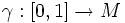$\gamma:[0,1] \to M$ be a smooth curve in$M$. Let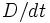$D/dt$ denote a connection along$\gamma$ of$E$. The transport along$\gamma$ defined by$D/dt$ maps$E_{\gamma(0)}$ to the space of sections of$E$ along$\gamma$, denoted in symbols as: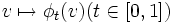$v \mapsto \phi_t(v) (t \in [0,1])$

such that for any vector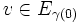$v \in E_{\gamma(0)}$: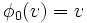$\phi_0(v) = v$

and$\frac{D (\phi_t(v))}{dt} = 0$

Intuitively, we define a rule for moving the fibre of$E$, in a manner that is parallel to itself with respect to the connection.

## Facts

### Connection gives transport

We saw above that a connection along a curve defines a rule of transport along that curve. Consider now a connection on the vector bundle. Then, for every curve, this gives rise to a connection along that curve, and hence a transport along that curve. Hence a connection gives a global rule for transporting frames.